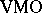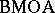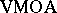# VMOA-space

space of analytic functions of vanishing mean oscillation

The class of analytic functions on the unit disc that are in(see also $\operatorname{BMO}$-space;-space;-space).

Fefferman's duality theorem (see $\operatorname{BMO}$-space) gives the characterization that an analytic function in $\operatorname{BMO}$ is inif and only if its boundary values can be expressed as the sum of a continuous function and the harmonic conjugate (cf. also Harmonic function) of a continuous function. This suggests that functions inare close to being continuous, but one has to be careful because their behaviour can be quite wild. For example, it can be show that any conformal mapping onto a region of finite area is in.

D. Sarason [a5] used the fact thatis the closure of the disc algebra $A$ into prove that $H ^ { \infty } + C$, with $C$ the class of continuous functions, is a closed subalgebra of $L^{\infty}$ and consequently the simplest example of a Douglas algebra (see-space).

The distance between a function $f$ inandhas attracted some interest, [a1], [a2], [a4]. Let $f$ be an analytic function on the unit disc, $\zeta$ a point on the boundary $T$ and write $K _ { \zeta }$ for the cluster set $\operatorname{Cl} ( f , \zeta )$. Using an assortment of tools from functional analysis, S. Axler and J. Shapiro [a1] proved that

\begin{equation*} \| f + \operatorname {VMOA} \| _ { * } \leq C \operatorname { lim sup } _ { \zeta \in T } \sqrt { \operatorname { area } ( K _ { \zeta } ) }. \end{equation*}

This led to a search for the optimal geometric condition for the right-hand side above, see [a4] for the answer.

How to Cite This Entry:
VMOA-space. Encyclopedia of Mathematics. URL: http://encyclopediaofmath.org/index.php?title=VMOA-space&oldid=50837
This article was adapted from an original article by D. Stegenga (originator), which appeared in Encyclopedia of Mathematics - ISBN 1402006098. See original article